Processing ......FreeComputerBooks.com Links to Free Computer, Mathematics, Technical Books all over the World

An Introduction to Measure Theory
Top Free Machine Learning Books 🌠 - 100% Free or Open Source!
• Title: An Introduction to Measure Theory
• Author(s) Terrence Tao
• Publisher: American Mathematical Society (AMS), 2011; eBook (Preliminary Version, 2012)
• Permission: Author's preliminary version made available with permission of the publisher, the AMS.
• Hardcover: 206 pages
• eBook: Author's Preliminary Version, PDF (265 pages, 1.3 MB)
• Language: English
• ISBN-10: 0821869191
• ISBN-13: 978-0821869192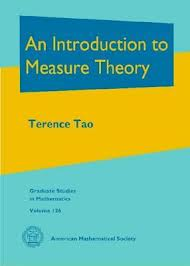Book Description

This is a graduate text introducing the fundamentals of measure theory and integration theory, which is the foundation of modern real analysis.

The text focuses first on the concrete setting of Lebesgue measure and the Lebesgue integral (which in turn is motivated by the more classical concepts of Jordan measure and the Riemann integral), before moving on to abstract measure and integration theory, including the standard convergence theorems, Fubini's theorem, and the Caratheodory extension theorem. Classical differentiation theorems, such as the Lebesgue and Rademacher differentiation theorems, are also covered, as are connections with probability theory.

The material is intended to cover a quarter or semester's worth of material for a first graduate course in real analysis. There is an emphasis in the text on tying together the abstract and the concrete sides of the subject, using the latter to illustrate and motivate the former.

The central role of key principles (such as Littlewood's three principles) as providing guiding intuition to the subject is also emphasized. There are a large number of exercises throughout that develop key aspects of the theory, and are thus an integral component of the text. As a supplementary section, a discussion of general problem-solving strategies in analysis is also given. The last three sections discuss optional topics related to the main matter of the book.

• Terrence Tao is an Australian-American mathematician who has worked in various areas of mathematics. He currently focuses on harmonic analysis, partial differential equations, algebraic combinatorics, arithmetic combinatorics, geometric combinatorics, compressed sensing and analytic number theory.
Reviews, Ratings, and Recommendations: Related Book Categories: Read and Download Links:Similar Books:
•Measure, Integration and Real Analysis (Sheldon Axler)

This textbook welcomes students into the fundamental theory of measure, integration, and real analysis. Focusing on an accessible approach, it lays the foundations for further study by promoting a deep understanding of key results.

•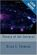Theory of the Integral (Brian S. Thomson)

This text is intended as a treatise for a rigorous course introducing the elements of integration theory on the real line. All of the important features of the Riemann integral, the Lebesgue integral, and the Henstock-Kurzweil integral are covered.

•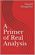A Primer of Real Analysis (Dan Sloughter)

This is a short introduction to the fundamentals of real analysis, written the text assuming the reader has the level of mathematical maturity of one who has completed the standard sequence of calculus courses.

•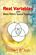Real Variables with Basic Metric Space Topology (Robert B. Ash)

Designed for a first course in real variables, this text presents the fundamentals for more advanced mathematical work, particularly in the areas of complex variables, measure theory, differential equations, functional analysis, and probability.

•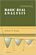Basic Real Analysis (Anthony W. Knapp)

This is a comprehensive treatment with a global view of the Real Analysis, emphasizing the connections between real analysis and other branches of mathematics. Included throughout are many examples and hundreds of problems.

•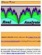Interactive Real Analysis (Bert G. Wachsmuth)

An interactive textbook for Real Analysis or Advanced Calculus in one real variable. It deals with sets, sequences, series, continuity, differentiability, integrability (Riemann and Lebesgue), topology, power series, and more.

•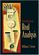Introduction to Real Analysis (William F. Trench)

Using an extremely clear and informal approach, this book introduces readers to a rigorous understanding of mathematical analysis and presents challenging math concepts as clearly as possible.

•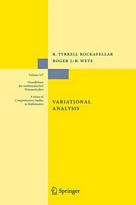Variational Analysis (R. Tyrrell Rockafellar, et al)

This book develops a unified framework and, in finite dimension, provides a detailed exposition of variational geometry and subdifferential calculus in their current forms beyond classical and convex analysis.

•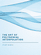The Art of Polynomial Interpolation (Stuart Murphy)

Exploring the techniques of interpolating data allows us to view the development and birth of a polynomial. This book is focused on laying a foundation for understanding and applying several common forms of Polynomial Interpolation.

Book Categories
 :All CategoriesTop Free BooksRecent BooksMiscellaneous BooksComputer EngineeringComputer LanguagesComputer ScienceData Science/DatabasesJava and Java EE (J2EE)Linux and UnixMathematicsMicrosoft and .NETMobile ComputingNetworking and CommunicationsSoftware EngineeringSpecial TopicsWeb Programming
Other Categories